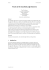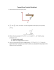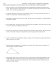# 15.1 Central Angles and Inscribed Angles

## Transcription

15.1 Central Angles and Inscribed Angles
```DO NOT EDIT--Changes must be made through “File info”
CorrectionKey=NL-A;CA-A
Name
Class
Date
15.1 Central Angles and
Inscribed Angles
Essential Question: How can you determine the measures of central angles and inscribed
angles of a circle?
Explore
Resource
Locker
Investigating Central Angles and
Inscribed Angles
A chord is a segment whose endpoints lie on a circle.
A central angle is an angle less than 180° whose vertex lies at the center of a circle.
An inscribed angle is an angle whose vertex lies on a circle and whose sides contain chords of
the circle.
The diagram shows two examples of an inscribed angle and the corresponding central angle.
Chords
Central Angle
A
A
_
_
AB and AB
B
C
B
∠ACD
Inscribed Angle
C
D
∠ACD
D
© Houghton Mifflin Harcourt Publishing Company
AUse a compass to draw a circle. Label the center C.
B
D
E
Use a straightedge to draw an acute inscribed angle on your circle
from Step A. Label the angle as ∠DEF.
C
Check students’ drawings.
CUse a straightedge to draw the corresponding central angle, ∠DCF.
F
Check students’ drawings.
D
Use a protractor to measure the inscribed angle and the central angle. Record the measure
of the inscribed angle, the measure of the central angle, and the measure of 360° minus the
central angle. List your results in the table.
Angle Measure
Circle C
Circle 2
Circle 3
Circle 4
Circle 5
Circle 6
Circle 7
m∠DEF
32°
51°
75°
90°
127°
155°
173°
m∠DCF
64°
102°
150°
180°
106°
50°
14°
360° - m∠DCF
296°
258°
210°
180°
254°
310°
346°
Module 15
GE_MNLESE385801_U6M15L1.indd 779
779
Lesson 1
02/04/14 11:30 AM
DO NOT EDIT--Changes must be made through “File info”
CorrectionKey=NL-A;CA-A
E
DO NOT
Correcti
Repeat Steps A-D six more times. Examine a variety of inscribed angles (two more acute,
one right, and three obtuse). Record your results in the table in Step D.
Answers may vary. Possible answers are given.
Reflect
1.
Examine the values in the first and second rows of the table. Is there a mathematical relationship that exists
for some or all of the values? Make a conjecture that summarizes your observation.
For inscribed angles with measures less than or equal to 90°, the measure of the inscribed
angle is equal to half the measure of the corresponding central angle.
2.
Examine the values in the first and third rows of the table. Is there a mathematical relationship that exists
for some or all of the values? Make a conjecture that summarizes your observation.
For inscribed angles with measures greater than or equal to 90°, the measure of the
inscribed angle is equal to half the difference between 360° and the measure of the
corresponding central angle.
Explain 1
Understanding Arcs and Arc Measure
An arc is a continuous portion of a circle consisting of two points (called the endpoints
of the arc) and all the points on the circle between them.
Arc
A minor arc is an arc whose
points are on or in the
interior of a corresponding
central angle.
Measure
Figure
The measure of a minor arc
is equal to the measure of
the central angle.
A
⁀ = m∠ACB
mAB
⁀
AB
C
B
The measure of a major
arc is equal to 360° minus
the measure of the central
angle.
⁀ = 360°-m∠ACB
A semicircle is an arc whose
endpoints are the endpoints
of a diameter.
The measure of a semicircle
is 180°.
© Houghton Mifflin Harcourt Publishing Company
A major arc is an arc whose
points are on or in the
exterior of a corresponding
central angle.
A
D
⁀
C
B
A
⁀
D
C
B
Module 15
GE_MNLESE385801_U6M15L1.indd 780
780
Lesson 1
22/03/14 11:17 PM
DO NOT EDIT--Changes must be made through “File info”
CorrectionKey=NL-A;CA-A
Adjacent arcs are arcs of the same circle that intersect in exactly one
⁀ and EF
⁀ are adjacent arcs.
point. DE
E
D
F
C
The measure of an arc formed by two adjacent arcs is the sum of
the measures of the two arcs.
⁀ +m DB
⁀
D
A
B
C
Example 1

⁀ using the
⁀ = 33°, determine m ABD
If m∠BCD = 18° and mEF
‹ ›
‹ ›
−
−
appropriate theorems and postulates. AF and BE intersect at Point C.
⁀ = 33°, then m∠ECF = 33°. If m∠ECF = 33°, then
Ifm EF
m∠ACB = 33° by the Vertical Angles Theorem. If m∠ACB = 33°
⁀ = 33° and mBD
⁀ = 18°. By the Arc
and m∠BCD = 18°, then mAB
⁀ =m AB
⁀ +m BD
⁀ = 51°.
⁀, and so mABD
A
B
E
C
F
⁀ = 27°, determine mNP
⁀ using the appropriate theorems and
JK
‹ ›
‹ ›
−
−
postulates. MK and NJ intersect at Point C.
D
© Houghton Mifflin Harcourt Publishing Company
Ifm
⁀ = 27°, then m∠JCK = 27°. If m∠JCK = 27°, then
JK
Ifm
m∠
MCN
= 27° by the Vertical Angles Theorem .
If m
⁀ = 27°
∠MCN = 27° and m∠MCP = 90 °, then mMN
andm
⁀ = 90 °. By the Arc Addition Postulate ,
MNP
m
M N
⁀ =m MN
⁀ +m NP
⁀, and so mNP = m
MNP
Module15
GE_MNLESE385801_U6M15L1.indd 781
⁀
MNP
781
P
C
J
K
⁀ = 63 °
- mMN
Lesson1
22/03/14 11:17 PM
DO NOT EDIT--Changes must be made through “File info”
CorrectionKey=NL-A;CA-A
DO NOT
Correcti
Reflect
3.
The minute hand of a clock sweeps out an arc as time moves forward. From 3:10 p.m.
to 3:30 p.m., what is the measure of this arc? Explain your reasoning.
11
12
1
2
10
6
2
3
9
8
4
7
1
10
3
9
8
12
11
5
4
7
3:10
6
5
3:30
1
A complete rotation corresponds to 60 minutes, so 20 minutes is __
of a complete rotation.
3
1
Since __
× 360° = 120°, the measure of the arc is 120°.
3
4.
⁀ = 45° and m∠ACD = 56°, determine mBD
⁀ using the appropriate
If mEF
‹ › −
‹ ›
‹ ›
−
−
theorems and postulates. AE , BF , and DC intersect at Point C.
D
E
F
B
C
A
⁀ = 45°, then m∠ECF = 45°. If m∠ECF = 45°, then m∠ACB = 45° by the Vertical
If mEF
⁀=
⁀ = 45° and mABD
Angles Theorem. If then m∠ACB = 45° and m∠ACD = 56°, then mAB
Explain 2
Using the Inscribed Angle Theorem
In the Explore you looked at the relationship
between central angles and inscribed angles.
Those results, combined with the definitions
of arc measure, lead to the following theorem
about inscribed angles and their intercepted arcs .
An intercepted arc consists of endpoints that lie
on the sides of an inscribed angle and all the
vpoints of the circle between them.
Inscribed Angle Theorem
The measure of an inscribed angle
is equal to half the measure
of its intercepted arc.
1 mAB
⁀
2
A
D
C
© Houghton Mifflin Harcourt Publishing Company
⁀ = mAB
⁀ + mBD
⁀ , and so mBD
⁀ = 11°.
56°. By the Arc Addition Postulate, mABD
B
Module 15
GE_MNLESE385801_U6M15L1.indd 782
782
Lesson 1
22/03/14 11:17 PM
DO NOT EDIT--Changes must be made through “File info”
CorrectionKey=NL-A;CA-A
Example 2 Use the Inscribed Angle Theorem to find inscribed angle measures.
⁀,m BD
⁀, m∠DAB, and m∠ADE using the appropriate
DE
theorems and postulates.
Determinem
By the Inscribed Angle Theorem,
B
18°
E
⁀, and so
m∠DAE = __12 mDE
⁀ = 2 × 54° = 108°. By the Arc Addition Postulate,
mDE
⁀ =m BE
⁀ +m ED
⁀ = 18°+ 108° = 126°. By the Inscribed Angle
mBD
C
54°
A
⁀ is a
⁀ = __1 × 126° = 63°. Note that ADE
Theorem, m∠DAB = __12 mBD
2
D
⁀ = 180°. By the Inscribed Angle Theorem,
semicircle, and so mADE
m∠ADE = __12 mADE = __12 × 180° = 90°.
⁀, m∠XWZ, and m∠WXZ using the appropriate
⁀,m XZ
WX
theorems and postulates.
Determinem
By the Inscribed Angle Theorem, m
and so m
therefore,m
X
W
⁀ is a semicircle and,
⁀ = 2 × 9° = 18° . Note that WXZ
WX
C
Z
9°
Y
106°
⁀ = 180°. By the Arc Addition Postulate ,
WXZ
⁀ =m WX
⁀ and then mXZ
⁀ = 180° - 18° = 162°
⁀ +m XZ
WXZ
m
By the,
Note that
Inscribed Angle Theorem m∠XWZ = __1 mXZ
⁀ = __1 × 162° = 81°.
2
2
⁀
WYZ
Angle Theorem, m
© Houghton Mifflin Harcourt Publishing Company
∠WZX =
_1 mWX,
2
⁀ = 180° . By the Inscribed
is a semicircle, and so mWYZ
∠WZX = __12 m
⁀
WYZ
= __12 × 180° = 90° .
Reflect
5.
⁀ in Example 2B.
DIscussion Explain an alternative method for determining m∠ XZ
Use the Inscribed Angle Theorem to determine m∠WXZ = 90°. The angle measures
in a triangle sum to 180°, therefore m∠XWZ = 81° and by the Inscribed Angles
Theorem, m⁀
XZ = 162°.
6.
Justify Reasoning How does the measure of ∠ABD compare to the
measure of ∠ACD? Explain your reasoning.
AD . Since the measure
m∠ABD = m∠ACD; Each angle intercepts ⁀
A
D
of each angle is equal to half the measure of ⁀
AD , the measures
of ∠ABD and ∠ACD are the same.
B
C
Module15
GE_MNLESE385801_U6M15L1.indd 783
783
Lesson1
22/03/14 11:17 PM
DO NOT EDIT--Changes must be made through “File info”
CorrectionKey=NL-A;CA-A
DO NOT
Correcti
7.
If m∠EDF = 15°, determine m∠ABE using the appropriate theorems and
postulates.
44°
A
Since ∠EDF and ∠EBF intercept the same arc, they have the same
F
C
measure. By the Inscribed Angle Theorem,
1 ⁀ __
m∠ABF = __
mAF = 12 × 44° = 22°. By the Angle Addition
2
E
B
Postulate,
D
m∠ABE = m∠ABF + m∠EBF. Therefore,
m∠ABE = 22° + 15° = 37°.
Explain 3
Investigating Inscribed Angles
on Diameters
You can examine angles that are inscribed in a semicircle. Example 3 Construct and
analyze an angle inscribed in a semicircle.
A
Use a compass to draw a circle with center C. Use a straightedge to draw a
_
diameter of the circle. Label the diameter DF.
B
Use a straightedge to draw an inscribed angle ∠DEF on your circle from Step
A whose sides contain the endpoints of the diameter.
E
D
F
C
Check students’ drawings.
C
Use a protractor to determine the measure of ∠DEF (to the nearest degree).
Record the results in the table.
Circle C
Circle 2
Circle 3
Circle 4
m∠DEF
90°
90°
90°
90°
D
Repeat the process three more times. Make sure to vary the size of the circle, and the
location of the vertex of the inscribed angle. Record the results in the table in Part C.
E
Examine the results, and make a conjecture about the measure of an angle inscribed in
a semicircle.
© Houghton Mifflin Harcourt Publishing Company
Angle Measure
The measure of an angle inscribed in a semicircle is 90°.
F
How can does the Inscribed Angle Theorem justify your conjecture?
The Inscribed Angle Theorem states that the measure of an inscribed angle is equal to
half the measure of its intercepted arc. The arc formed by a diameter is a semicircle with a
measure equal to 180°. Therefore, the measure of the inscribed angle is 90°.
Module 15
GE_MNLESE385801_U6M15L1.indd 784
784
Lesson 1
22/03/14 11:17 PM
DO NOT EDIT--Changes must be made through “File info”
CorrectionKey=NL-A;CA-A
Inscribed Angle of a Diameter Theorem
The endpoints of a diameter lie on an inscribed
angle if and only if the inscribed angle is a right angle.
Reflect
8.
A right angle is inscribed in a circle. If the endpoints of its intercepted arc are connected by a segment,
must the segment pass through the center of the circle?
Yes, the endpoints of the intercepted arc lie on a diameter. And by definition, a diameter
passes through the center of the circle.
Elaborate
9.
An equilateral triangle is inscribed in a circle. How does the relationship
between the measures of the inscribed angles and intercepted arcs help
determine the measure of each angle of the triangle?
© Houghton Mifflin Harcourt Publishing Company
Together the three angles intercept the entire circle, which has a measure of 360°. Since
the angles of an equilateral triangle are congruent, they each intercept an arc of 120°. The
measure of an inscribed angle is equal to half the measure of its intercepted arc, so each
angle of the triangle measures 60°.
10. Essential Question Check-In What is the relationship between inscribed
angles and central angles in a circle?
If an inscribed angle is acute, its measure is equal to half the measure of its associated
central angle. If an inscribed angle is obtuse, its measure is equal to half the difference
between the measure of its associated central angle and 360°.
Module 15
GE_MNLESE385801_U6M15L1.indd 785
785
Lesson 1
22/03/14 11:17 PM
```

### Review12.1-12.6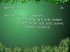### If ABC is obtuse, what are the restrictions on x? ∠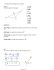### installation of an angle board * * * thermopian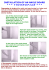### Circle Geometry Practice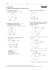### 10.5 Practice A & B Worksheet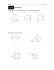### Unit 8: Circle Geometry Grade 9 Math Introduction: Definitions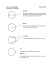### square, circle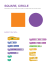### Proofs of the inscribed angle theorem Pavel Leischner